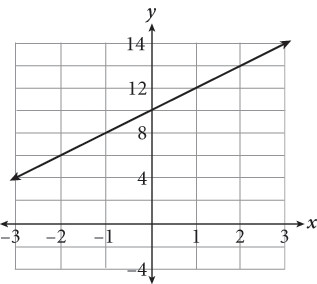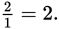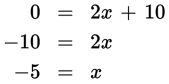# SAT Math Multiple Choice Question 543: Answer and Explanation

### Test Information

Question: 543

3.Where will the line shown in the graph above intersect the x-axis?

• A. –5.5
• B. –5
• C. –4.5
• D. –4

Explanation:

B

Difficulty: Easy

Category: Heart of Algebra / Linear Equations

Strategic Advice: Finding an x-intercept is easy when you know the equation of the line—it's the value of x when y is 0. Notice that the answer choices are very close together. This means you shouldn't just estimate visually. Take the time to do the math.

Getting to the Answer: Everything you need to write the equation is shown on the graph—just pay careful attention to how the grid-lines are labeled. The y-intercept is 10 and the line rises 2 units and runs 1 unit from one point to the next, so the slope isThis means the equation of the line, in slope-intercept form, is y = 2x + 10. Now, set the equation equal to zero and solve for x:The line will intersect the x-axis at –5.